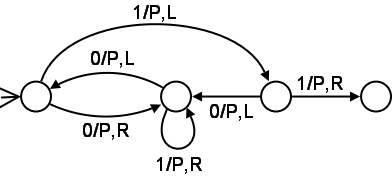LMU COMPUTER SCIENCE
Using LaTeX

Many classes will have assignments that require problem solutions to be submitted in some format (such as PDF or PostScript) generated from LaTeX source. It is actually a Good Thing to have standards for homework submissions — it helps the graders find the solutions quickly, and it helps the students get used to following directions, which is, after all, a trait that many employers dearly love. These notes cover our standard submission format.

Help with LaTeX can be found by reading this introduction (give yourself a couple hours... it will be well worth it: you can't just guess at how to do LaTeX well).

## Submission Guidelines

Finished? Good. Now let's get right to the standardized homework submission guidelines. Create a file called test.tex with the following contents:

\documentclass[11pt]{article}
\usepackage{fullpage}
\title{CMSI 101 Homework \#3}
\author{Yukihiro Matsumoto and Donald Knuth}
\begin{document}
\maketitle
\begin{enumerate}
\item I think that $\sum_{k=1}^{n} n = \frac{n(n-1)}{2}$
\item I'm not sure what the answer'' to this problem is \ldots.  But
it may have something to do with the fact that $a^{\log b} = b^{\log a}$
or maybe not.
\item This is a JavaScript function to square its argument
\begin{verbatim}
function square(x) {
return x * x;
}
\end{verbatim}
\item $\Omega(n^2) = \{f\:|\:\exists k\:N.\;\forall n \ge N.\;kn^2 \le f(n)\}$
\item I've \emph{never} been to Waik\=ik\=i
\item Sometimes you have to show the equation on another line
\begin{displaymath}
\sum_{k=1}^{n} n = \frac{n(n-1)}{2}
\end{displaymath}
\item You can style words like \texttt{you are coding} or
\textsf{without serifs}.
\item See the attached file \texttt{circle.rb}.
\item L\'a fh\'eile P\'adraig sona daoibh. $\lambda x. e^{x/2}$
\end{enumerate}
\end{document}


Then run the command

pdflatex test.tex


and you will have a nice PDF file.

Do you like it? I hope so. Now remember to make all your homework solution documents look like this.

## Images

Generally, a crappy pencil-drawn sketch on a ripped piece of paper taped to the rest of the pages of your homework submission may cause you to lose points. Make sure you learn how to use a drawing program and export images and include them in your LaTeX documents.

If you'd like to try it now, save this image as bb.png.Then save the following as homework.tex:

\documentclass[11pt]{article}
\usepackage{fullpage}
\usepackage{graphicx}
\title{CMSI 101 Homework \#5}
\author{Alan Turing}
\begin{document}
\maketitle
\begin{enumerate}
\item This is something cool:
\begin{center}
\includegraphics[width=3in]{bb.png}
\end{center}
\item I think that the Lambda Calculus is as powerful as any
finite state computing device.
\end{enumerate}
\end{document}


Run pdflatex as before and admire your result.

## Writing Actual Papers

LaTeX is a professional typesetting system and thus allows you to create pristine, camera-ready papers, articles, reports, and books. It completely automates tasks like footnoting, making bibliographies, indexing, producing tables of contents, and so on..., keeping all references in sync. Producing bibliographies automatically is reason enough to use the tool: for one thing, you need only list the components of the source (i.e., the author(s), titles, publication date, etc.) and LaTeX will perfectly format the bibliographic entry for you. Never again will you lose points on your papers for sloppy bibliographies.

Do yourself a huge favor by reading Dr. Dionisio's Annotated LaTeX Guide for almost everything you need to know about constructing a great (-looking) paper. Tip: Use LaTeX for all your papers, not just for those in your computer science classes. They are practically guaranteed to look better than the sorry MS-Word efforts from your friends in the liberal arts.